## 长短期记忆网络

LSTM 网络是一种循环神经网络 (RNN)，可以学习序列数据的时间步之间的长期依存关系。

### LSTM 网络架构

LSTM 网络的核心组件是序列输入层和 LSTM 层。序列输入层将序列或时间序列数据输入网络中。LSTM 层学习序列数据的时间步之间的长期相关性。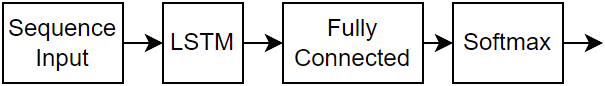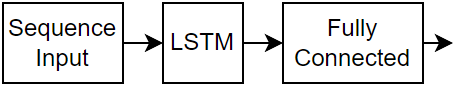#### 分类 LSTM 网络

```numFeatures = 12; numHiddenUnits = 100; numClasses = 9; layers = [ ... sequenceInputLayer(numFeatures) lstmLayer(numHiddenUnits,'OutputMode','last') fullyConnectedLayer(numClasses) softmaxLayer classificationLayer];```

```numFeatures = 12; numHiddenUnits = 100; numClasses = 9; layers = [ ... sequenceInputLayer(numFeatures) lstmLayer(numHiddenUnits,'OutputMode','sequence') fullyConnectedLayer(numClasses) softmaxLayer classificationLayer];```

#### 回归 LSTM 网络

```numFeatures = 12; numHiddenUnits = 125; numResponses = 1; layers = [ ... sequenceInputLayer(numFeatures) lstmLayer(numHiddenUnits,'OutputMode','last') fullyConnectedLayer(numResponses) regressionLayer];```

```numFeatures = 12; numHiddenUnits = 125; numResponses = 1; layers = [ ... sequenceInputLayer(numFeatures) lstmLayer(numHiddenUnits,'OutputMode','sequence') fullyConnectedLayer(numResponses) regressionLayer];```

#### 视频分类网络

```inputSize = [28 28 1]; filterSize = 5; numFilters = 20; numHiddenUnits = 200; numClasses = 10; layers = [ ... sequenceInputLayer(inputSize,'Name','input') sequenceFoldingLayer('Name','fold') convolution2dLayer(filterSize,numFilters,'Name','conv') batchNormalizationLayer('Name','bn') reluLayer('Name','relu') sequenceUnfoldingLayer('Name','unfold') flattenLayer('Name','flatten') lstmLayer(numHiddenUnits,'OutputMode','last','Name','lstm') fullyConnectedLayer(numClasses, 'Name','fc') softmaxLayer('Name','softmax') classificationLayer('Name','classification')];```

```lgraph = layerGraph(layers); lgraph = connectLayers(lgraph,'fold/miniBatchSize','unfold/miniBatchSize');```

#### 更深的 LSTM 网络

```numFeatures = 12; numHiddenUnits1 = 125; numHiddenUnits2 = 100; numClasses = 9; layers = [ ... sequenceInputLayer(numFeatures) lstmLayer(numHiddenUnits1,'OutputMode','sequence') dropoutLayer(0.2) lstmLayer(numHiddenUnits2,'OutputMode','last') dropoutLayer(0.2) fullyConnectedLayer(numClasses) softmaxLayer classificationLayer];```

```numFeatures = 12; numHiddenUnits1 = 125; numHiddenUnits2 = 100; numClasses = 9; layers = [ ... sequenceInputLayer(numFeatures) lstmLayer(numHiddenUnits1,'OutputMode','sequence') dropoutLayer(0.2) lstmLayer(numHiddenUnits2,'OutputMode','sequence') dropoutLayer(0.2) fullyConnectedLayer(numClasses) softmaxLayer classificationLayer];```

### 网络层`sequenceInputLayer`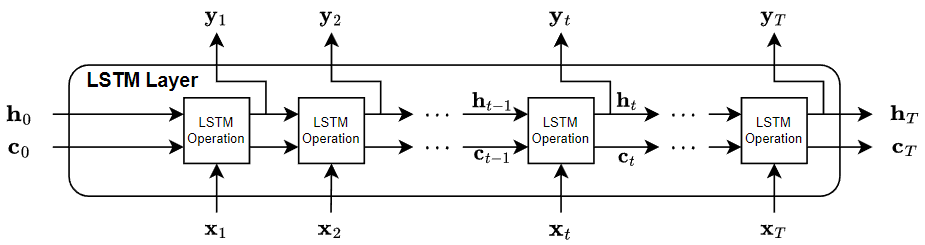`lstmLayer`

LSTM 层学习时间序列和序列数据中时间步之间的长期相关性。`bilstmLayer``gruLayer`

GRU 层学习时间序列和序列数据中时间步之间的相关性。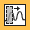`convolution1dLayer``maxPooling1dLayer``averagePooling1dLayer``globalMaxPooling1dLayer``sequenceFoldingLayer``sequenceUnfoldingLayer``flattenLayer``wordEmbeddingLayer` (Text Analytics Toolbox)

### 分类、预测和预报

LSTM 网络可以记住各次预测之间的网络状态。如果您事先没有完整的时间序列，或您要对一个长时间序列进行多次预测，则网络状态会很有用。

### 序列填充、截断和拆分

LSTM 网络支持具有不同序列长度的输入数据。当使数据通过网络时，软件会填充、截断或拆分序列，以便每个小批量中的所有序列都具有指定的长度。您可以使用 `trainingOptions` 中的 `SequenceLength``SequencePaddingValue` 名称-值对组参数来指定序列长度和用于填充序列的值。

#### 按长度对序列排序

```sequenceLengths = cellfun(@(X) size(X,2), XTrain); [sequenceLengthsSorted,idx] = sort(sequenceLengths); XTrain = XTrain(idx);```#### 填充序列#### 截断序列#### 拆分序列#### 指定填充方向### 归一化序列数据

```mu = mean([XTrain{:}],2); sigma = std([XTrain{:}],0,2); XTrain = cellfun(@(X) (X-mu)./sigma,XTrain,'UniformOutput',false);```

### LSTM 层架构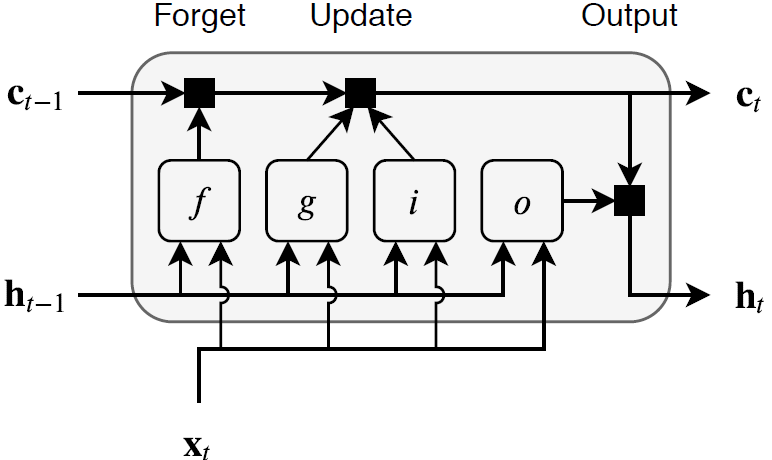LSTM 层的可学习权重包括输入权重 W (`InputWeights`)、循环权重 R (`RecurrentWeights`) 以及偏置 b (`Bias`)。矩阵 W、R 和 b 分别是输入权重、循环权重和每个分量的偏置的串联。这些矩阵的串联如下：

`$W=\left[\begin{array}{c}{W}_{i}\\ {W}_{f}\\ {W}_{g}\\ {W}_{o}\end{array}\right],R=\left[\begin{array}{c}{R}_{i}\\ {R}_{f}\\ {R}_{g}\\ {R}_{o}\end{array}\right],b=\left[\begin{array}{c}{b}_{i}\\ {b}_{f}\\ {b}_{g}\\ {b}_{o}\end{array}\right],$`

`${c}_{t}={f}_{t}\odot {c}_{t-1}+{i}_{t}\odot {g}_{t},$`

`${h}_{t}={o}_{t}\odot {\sigma }_{c}\left({c}_{t}\right),$`

 Hochreiter, S., and J. Schmidhuber. "Long short-term memory." Neural computation. Vol. 9, Number 8, 1997, pp.1735–1780.